# Program to implement Logic Gates

A Logic gate is an elementary building block of any digital circuits. It takes one or two inputs and produces output based on those inputs. Outputs may be high (1) or low (0). Logic gates are implemented using diodes or transistors. It can also be constructed using vacuum tubes, electromagnetic elements like optics, molecule etc. In a computer, most of the electronic circuits are made up logic gates. Logic gates are used to create a circuit that performs calculations, data storage or shows off object-oriented programming especially the power of inheritance.

There are seven basic logic gates defined, these are:

1. AND gate,
2. OR gate,
3. NOT gate,
4. NAND gate,
5. NOR gate,
6. XOR gate and
7. XNOR gate.

Below are the brief details about them along with their implementation:

1. AND Gate
The AND gate gives an output of 1 if both the two inputs are 1, it gives 0 otherwise.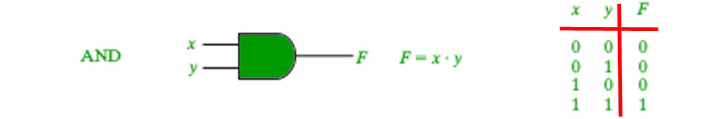Below are the programs to implement AND gate using various methods:

1. Using product method.
2. Using if else condition.
3. Using “and (&)” operator.

## Product Method

 `// C program implementing the AND gate ` `// through product method. ` ` `  `#include ` `#include ` ` `  `int` `main() ` `{ ` `    ``int` `a = { 1, 0, 1, 0, 1 }; ` `    ``int` `b = { 0, 1, 1, 0, 0 }; ` `    ``int` `i, product; ` ` `  `    ``for` `(i = 0; i < 5; i++) { ` ` `  `        ``// using product method ` `        ``product = a[i] * b[i]; ` ` `  `        ``printf``(``"\n %d AND %d = %d"``, ` `               ``a[i], b[i], product); ` `    ``} ` `} `

## & Operator

 `// C program implementing the AND gate ` `// using & operator ` ` `  `#include ` `#include ` ` `  `int` `main() ` `{ ` `    ``int` `a = { 1, 0, 1, 0, 1 }; ` `    ``int` `b = { 0, 1, 1, 0, 0 }; ` `    ``int` `i, and_ans; ` ` `  `    ``for` `(i = 0; i < 5; i++) { ` ` `  `        ``// using the & operator ` `        ``and_ans = a[i] & b[i]; ` ` `  `        ``printf``(``"\n %d AND %d = %d"``, ` `               ``a[i], b[i], and_ans); ` `    ``} ` `} `

## If-Else

 `// C program implementing the AND gate ` `// using if and else condition ` ` `  `#include ` `#include ` ` `  `int` `main() ` `{ ` `    ``int` `a = { 1, 0, 1, 0, 1 }; ` `    ``int` `b = { 0, 1, 1, 0, 0 }; ` `    ``int` `i, ans; ` ` `  `    ``for` `(i = 0; i < 5; i++) { ` `        ``if` `(a[i] == 0 && b[i] == 0) ` `            ``ans = 0; ` ` `  `        ``else` `if` `(a[i] == 0 && b[i] == 1) ` `            ``ans = 0; ` ` `  `        ``else` `if` `(a[i] == 1 && b[i] == 0) ` `            ``ans = 0; ` `        ``else` `            ``ans = 1; ` ` `  `        ``printf``(``"\n %d AND %d = %d"``, ` `               ``a[i], b[i], ans); ` `    ``} ` `} `

Output:

```1 AND 0 = 0
0 AND 1 = 0
1 AND 1 = 1
0 AND 0 = 0
1 AND 0 = 0
```
2. OR Gate
The OR gate gives an output of 1 if either of the two inputs are 1, it gives 0 otherwise.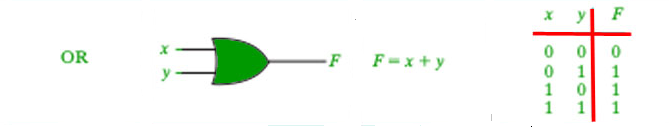Below are the programs to implement AND gate using various methods:

1. Using + operator.
2. Using | operator.
3. Using || operator.
4. Using if else.

## + Operator

 `// C program implementing the OR gate ` `// using + operator ` ` `  `#include ` `#include ` ` `  `int` `main() ` `{ ` `    ``int` `a = { 1, 0, 1, 0, 1 }; ` `    ``int` `b = { 0, 1, 1, 0, 0 }; ` `    ``int` `i, or_ans; ` ` `  `    ``for` `(i = 0; i < 5; i++) { ` ` `  `        ``// using the + operator ` `        ``if` `(a[i] + b[i] > 0) ` `            ``or_ans = 1; ` `        ``else` `            ``or_ans = 0; ` ` `  `        ``printf``(``"\n %d AND %d = %d"``, ` `               ``a[i], b[i], or_ans); ` `    ``} ` `} `

## | Operator

 `// C program implementing the OR gate ` `// using | operator ` ` `  `#include ` `#include ` ` `  `int` `main() ` `{ ` `    ``int` `a = { 1, 0, 1, 0, 1 }; ` `    ``int` `b = { 0, 1, 1, 0, 0 }; ` `    ``int` `i, or_ans; ` ` `  `    ``for` `(i = 0; i < 5; i++) { ` ` `  `        ``// using the | operator ` `        ``or_ans = a[i] | b[i]; ` ` `  `        ``printf``(``"\n %d AND %d = %d"``, ` `               ``a[i], b[i], or_ans); ` `    ``} ` `} `

## || Operator

 `// C program implementing the OR gate ` `// using || operator ` ` `  `#include ` `#include ` ` `  `int` `main() ` `{ ` `    ``int` `a = { 1, 0, 1, 0, 1 }; ` `    ``int` `b = { 0, 1, 1, 0, 0 }; ` `    ``int` `i, or_ans; ` ` `  `    ``for` `(i = 0; i < 5; i++) { ` ` `  `        ``// using the || operator ` `        ``or_ans = a[i] || b[i]; ` ` `  `        ``printf``(``"\n %d AND %d = %d"``, ` `               ``a[i], b[i], or_ans); ` `    ``} ` `} `

## If-Else

 `// C program implementing the OR gate ` `// using if else ` ` `  `#include ` `#include ` ` `  `int` `main() ` `{ ` `    ``int` `a = { 1, 0, 1, 0, 1 }; ` `    ``int` `b = { 0, 1, 1, 0, 0 }; ` `    ``int` `i, or_ans; ` ` `  `    ``for` `(i = 0; i < 5; i++) { ` ` `  `        ``// using the if-else conditions ` `        ``if` `(a[i] == 0 && b[i] == 0) ` `            ``or_ans = 0; ` `        ``else` `            ``or_ans = 1; ` ` `  `        ``printf``(``"\n %d AND %d = %d"``, ` `               ``a[i], b[i], or_ans); ` `    ``} ` `} `

Output:

```1 AND 0 = 1
0 AND 1 = 1
1 AND 1 = 1
0 AND 0 = 0
1 AND 0 = 1
```
3. NAND Gate
The NAND gate (negated AND) gives an output of 0 if both inputs are 1, it gives 1 otherwise.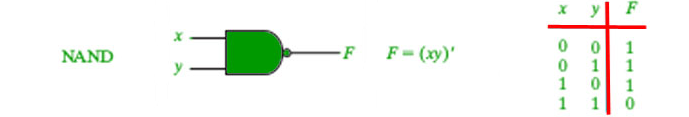Below are the programs to implement NAND gate using various methods:

1. Using if else.
2. Using Complement of the product.

## If-Else

 `// C program implementing the NAND gate ` ` `  `#include ` `#include ` ` `  `int` `main() ` `{ ` ` `  `    ``int` `a = { 1, 0, 1, 0, 1 }; ` `    ``int` `b = { 0, 1, 1, 0, 0 }; ` `    ``int` `i, ans; ` ` `  `    ``for` `(i = 0; i < 5; i++) { ` `        ``if` `(a[i] == 1 && b[i] == 1) ` `            ``ans = 0; ` `        ``else` `            ``ans = 1; ` `        ``printf``(``"\n %d NAND %d = %d"``, ` `               ``a[i], b[i], ans); ` `    ``} ` `} `

## Complement of the product

 `// C program implementing the NAND gate ` ` `  `#include ` `#include ` ` `  `int` `main() ` `{ ` ` `  `    ``int` `a = { 1, 0, 1, 0, 1 }; ` `    ``int` `b = { 0, 1, 1, 0, 0 }; ` `    ``int` `i, ans; ` ` `  `    ``for` `(i = 0; i < 5; i++) { ` `        ``ans = !(a[i] * b[i]); ` `        ``printf``(``"\n %d NAND %d = %d"``, ` `               ``a[i], b[i], ans); ` `    ``} ` `} `

Output:

```1 NAND 0 = 1
0 NAND 1 = 1
1 NAND 1 = 0
0 NAND 0 = 1
1 NAND 0 = 1
```
4. NOR Gate
The NOR gate (negated OR) gives an output of 1 if both inputs are 0, it gives 1 otherwise.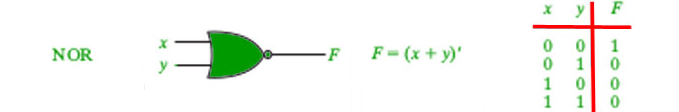Below are the programs to implement NOR gate using various methods:

1. Using + Operator.
2. Using if else.

## + Operator

 `// C program implementing the NOR gate ` ` `  `#include ` `#include ` ` `  `int` `main() ` `{ ` ` `  `    ``int` `a = { 1, 0, 1, 0, 1 }; ` `    ``int` `b = { 0, 1, 1, 0, 0 }; ` `    ``int` `i, ans; ` ` `  `    ``for` `(i = 0; i < 5; i++) { ` `        ``ans = !(a[i] + b[i]); ` `        ``printf``(``"\n %d NOR %d = %d"``, ` `               ``a[i], b[i], ans); ` `    ``} ` `} `

## If-Else

 `// C program implementing the NOR gate ` ` `  `#include ` `#include ` ` `  `int` `main() ` `{ ` ` `  `    ``int` `a = { 1, 0, 1, 0, 1 }; ` `    ``int` `b = { 0, 1, 1, 0, 0 }; ` `    ``int` `i, ans; ` ` `  `    ``for` `(i = 0; i < 5; i++) { ` `        ``if` `(a[i] == 0 && b[i] == 0) ` `            ``ans = 1; ` `        ``else` `            ``ans = 0; ` `        ``printf``(``"\n %d NOR %d = %d"``, ` `               ``a[i], b[i], ans); ` `    ``} ` `} `

Output:

```1 NOR 0 = 0
0 NOR 1 = 0
1 NOR 1 = 0
0 NOR 0 = 1
1 NOR 0 = 0
```
5. NOT Gate

It acts as an inverter. It takes only one input. If the input is given as 1, it will invert the result as 0 and vice-versa.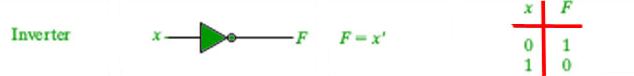Below are the programs to implement NOT gate using various methods:

1. Using ! Operator.
2. Using if else.

## If-Else

 `// C program implementing the NOT gate ` ` `  `#include ` `#include ` ` `  `int` `main() ` `{ ` ` `  `    ``int` `a = { 1, 0, 1, 0, 1 }; ` `    ``int` `i, ans; ` ` `  `    ``for` `(i = 0; i < 5; i++) { ` `        ``if` `(a[i] == 0) ` `            ``ans = 1; ` `        ``else` `            ``ans = 0; ` `        ``printf``(``"\n  NOT %d = %d"``, a[i], ans); ` `    ``} ` `} `

## ! Operator

 `// C program implementing the NOT gate ` ` `  `#include ` `#include ` ` `  `int` `main() ` `{ ` ` `  `    ``int` `a = { 1, 0, 1, 0, 1 }; ` `    ``int` `i, ans; ` ` `  `    ``for` `(i = 0; i < 5; i++) { ` `        ``ans = !(a[i]); ` `        ``printf``(``"\n  NOT %d = %d"``, a[i], ans); ` `    ``} ` `} `

Output:

```NOT 1 = 0
NOT 0 = 1
NOT 1 = 0
NOT 0 = 1
NOT 1 = 0
```

Attention reader! Don’t stop learning now. Get hold of all the important CS Theory concepts for SDE interviews with the CS Theory Course at a student-friendly price and become industry ready.

My Personal Notes arrow_drop_upTalk is cheap Show me the code -)

If you like GeeksforGeeks and would like to contribute, you can also write an article using contribute.geeksforgeeks.org or mail your article to contribute@geeksforgeeks.org. See your article appearing on the GeeksforGeeks main page and help other Geeks.

Please Improve this article if you find anything incorrect by clicking on the "Improve Article" button below.